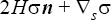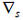# SURFACE_TENSION_MODEL

AcuSolve Command

## Syntax

SURFACE_TENSTION_MODEL("name") {parameters...}

User-given name.

## Parameters

type (enumerated) [=none]
Type of the surface tension.
constant or const
Constant surface tension. Requires surface_tension.
piecewise_linear or linear
Piecewise linear curve fit. Requires curve_fit_values and curve_fit_variable.
cubic_spline or spline
Cubic spline curve fit. Requires curve_fit_values and curve_fit_variable.
user_function or user
User-defined function. Requires user_function, user_values and user_strings.
surface_tension (real) >=0 [=0]
Constant value of the surface tension. Used with constant type.
curve_fit_values or curve_values (array) [={0,0}]
A two-column array of independent-variable/surface tension data values. Used with piecewise_linear and cubic_spline types.
curve_fit_variable or curve_var (enumerated) [=temperature]
Independent variable of the curve fit. Used with piecewise_linear and cubic_spline types.
temperature or temp
Temperature.
species_1 or spec1
Species 1.
species_2 or spec2
Species 2.
species_3 or spec3
Species 3.
species_4 or spec4
Species 4.
species_5 or spec5
Species 5.
species_6 or spec6
Species 6.
species_7 or spec7
Species 7.
species_8 or spec8
Species 8.
species_9 or spec9
Species 9.
user_function or user (string) [no default]
Name of the user-defined function. Used with user_function type.
user_values (array) [={}]
Array of values to be passed to the user-defined function. Used with user_function type.
user_strings (list) [={}]
Array of strings to be passed to the user-defined function. Used with user_function type.
multiplier_function (string) [=none]
User-given name of the multiplier function for scaling the viscosity. If none, no scaling is performed.

## Description

Surface tension adds a traction to a free surface of the form(1)where H is the surface curvature, n is the outward normal,is the surface gradient, and $\sigma$ is the surface tension defined by this command. The formulation used in AcuSolve removes the necessity of computing the surface curvature and the surface gradient of the surface tension through an integration by parts procedure. However, the contact angle then explicitly appears, which is modeled separately. See the CONTACT_ANGLE_MODEL command. For example, a constant surface tension may be defined by:
SURFACE_TENSION_MODEL( "air/water" ) {
type                                = constant
surface_tension                     = 0.07
}
SURFACE_TENSION_MODEL commands are referenced by SIMPLE_BOUNDARY_CONDITION and FREE_SURFACE commands, for example:
SIMPLE_BOUNDARY_CONDITION( "free surface" ) {
shape                 = three_node_triangle
element_set           = "water"
type                  = free_surface
surface_tension_model = "water/air"
contact_angle_model   = "water/air @ smooth aluminum"
}
and
FREE_SURFACE( "free surface" ) {
shape                  = three_node_triangle
element_set            = "water"
surface_tension_model  = "water/air"
contact_angle_model    = "water/air @ smooth aluminum"
}
Surface tension models of types piecewise_linear and cubic_spline may be used to define surface tension as a function of a single independent variable. For example,
SURFACE_TENSION_MODEL( "curve fit surface tension model" ) {
type                = piecewise_linear
curve_fit_values    = { 273, 0.07 ;
323, 0.07 ;
373, 0.06 ;
423, 0.05 ; }
curve_fit_variable   = temperature
}

defines surface tension as a function of temperature. In general, the problem must contain the variable defined by curve_fit_variable. The curve_fit_values parameter is a two-column array corresponding to the independent variable and the surface tension values. The independent variable values must be in ascending order. The limit point values of the curve fit are used when curve_fit_variable falls outside of the curve fit limits.

The curve_fit_values data may be read from a file. For the above example, the curve fit values may be placed in a file, such as surface_tension.fit:
273      0.07
323      0.07
373      0.06
423      0.05
SURFACE_TENSION_MODEL( "curve fit surface tension model" ) {
type                = piecewise_linear
curve_fit_variable  = temperature
}

A surface tension model of type user_function may be used to model more complex behaviors; see the AcuSolve User-Defined Functions Manual for a detailed description of user-defined functions.

The multiplier_function parameter may be used to uniformly scale the surface tension values. The value of this parameter refers to the user-given name of a MULTIPLIER_FUNCTION command in the input file. For example, a ramped surface tension may be specified by:
SURFACE_TENSION_MODEL( "curve fit surface tension model" ) {
type               = piecewise_linear
}